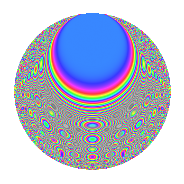# Properties

 Label 504.2.csLevel 504 Weight 2 Character orbit cs Rep. character $$\chi_{504}(85,\cdot)$$ Character field $$\Q(\zeta_{6})$$ Dimension 144 Newform subspaces 2 Sturm bound 192 Trace bound 6

# Related objects

## Defining parameters

 Level: $$N$$ = $$504 = 2^{3} \cdot 3^{2} \cdot 7$$ Weight: $$k$$ = $$2$$ Character orbit: $$[\chi]$$ = 504.cs (of order $$6$$ and degree $$2$$) Character conductor: $$\operatorname{cond}(\chi)$$ = $$72$$ Character field: $$\Q(\zeta_{6})$$ Newform subspaces: $$2$$ Sturm bound: $$192$$ Trace bound: $$6$$ Distinguishing $$T_p$$: $$5$$

## Dimensions

The following table gives the dimensions of various subspaces of $$M_{2}(504, [\chi])$$.

Total New Old
Modular forms 200 144 56
Cusp forms 184 144 40
Eisenstein series 16 0 16

## Trace form

 $$144q - 6q^{6} + 12q^{8} + O(q^{10})$$ $$144q - 6q^{6} + 12q^{8} + 12q^{12} - 22q^{18} + 14q^{20} + 24q^{23} - 2q^{24} + 72q^{25} - 56q^{26} - 56q^{30} - 10q^{32} + 16q^{33} + 12q^{34} - 28q^{38} - 24q^{39} + 12q^{40} - 8q^{41} - 20q^{42} - 64q^{44} + 24q^{46} - 24q^{48} - 72q^{49} + 46q^{50} - 18q^{52} + 42q^{54} + 8q^{57} + 18q^{58} - 16q^{60} + 48q^{62} - 60q^{64} + 118q^{66} + 78q^{68} - 112q^{71} + 34q^{72} - 12q^{76} + 8q^{78} + 88q^{80} - 8q^{81} - 36q^{82} + 14q^{84} - 42q^{86} - 104q^{87} - 24q^{88} - 160q^{90} + 66q^{92} + 6q^{94} - 64q^{95} - 24q^{96} + O(q^{100})$$

## Decomposition of $$S_{2}^{\mathrm{new}}(504, [\chi])$$ into newform subspaces

Label Dim. $$A$$ Field CM Traces $q$-expansion
$$a_2$$ $$a_3$$ $$a_5$$ $$a_7$$
504.2.cs.a $$72$$ $$4.024$$ None $$0$$ $$0$$ $$0$$ $$36$$
504.2.cs.b $$72$$ $$4.024$$ None $$0$$ $$0$$ $$0$$ $$-36$$

## Decomposition of $$S_{2}^{\mathrm{old}}(504, [\chi])$$ into lower level spaces

$$S_{2}^{\mathrm{old}}(504, [\chi]) \cong$$ $$S_{2}^{\mathrm{new}}(72, [\chi])$$$$^{\oplus 2}$$

## Hecke Characteristic Polynomials

There are no characteristic polynomials of Hecke operators in the database